Mobile QR Code1. (Sri Sivasubramaniya Nadar College of Engineering (SSN), Chennai, India )

Elliptic curve cryptography, Karatsuba, Vedic, multipliers, National Institute of Standards and Technology

## I. INTRODUCTION

Finite field multiplication is being used in many applications like cryptography, signal processing, etc. as they compose the key components in arithmetic operations. In the case of ECC, scalar multiplication is the most important operation which is done using finite field multiplication. For the elliptic curve implementation, The National Institute of Standards and Technology (NIST) has recommended five binary extension fields, i.e., m = 163, 233, 283, 409, and 571. Point multiplication (scalar multiplication) kP, where k is an integer and P is a point on the curve, is done using point addition and point doubling operation, both of which can be realized by affine or projective coordinates.

Many researchers have implemented the optimized ECC algorithm in hardware. Their main focus for optimization is generally on the efficient utilization of arithmetic operations, registers, or the multiplier that is used for the scalar multiplication operation. The authors in  have presented the implementation of ECC using the Nikhilam, Karatsuba, and Urdhva tirakbhyam algorithms for repeated multiplication operations. They have developed a hybrid multiplier algorithm by combining the Overlap-Free Karatsuba Algorithm (OFKA) with a block recombination approach (OFKABR) to improve the complexity of the original OFKA. The hybrid multiplier algorithms were compared in terms of time delay, resources utilized, and power consumption and were found to be efficient over a finite field GF(2$^{m}$). In , an area-efficient and high-speed FPGA implementation of scalar multiplication employing a Vedic multiplier which showed efficient results compared to the Karatsuba multiplication algorithm is presented. In 2007, Chester Rebeiro et al.  proposed an in-depth study on the implementation of the hybrid Karatsuba multiplier for GF (2$^{m}$). They have indeed shown a completely unique masking technique to avert the multiplier from power-based side-channel attacks and their work is found to be area efficient. In , a core multiplier to construct an efficient sequential polynomial multiplier based on the known iterative Karatsuba method is used. The authors in  have proposed three methods like a novel KA-based approach, an efficient register minimization, and an efficient FPGA-specific digit-parallel implementation strategy. In the case of Three-Operation Multiplication (TOM) over binary extension field, a novel approach is followed in  by mapping an efficient KA-based algorithm into a novel digit-serial multiplier and obtaining a new TOM structure through the novel derivation of the TOM algorithm which is found to have lower area-time-complexities when compared with the existing TOMs. The authors in  developed a high-performance ECC encryption system by proposing a Vedic multiplier architecture. The proposed multiplier is encoded and has only shift registers and adder circuits thus reducing the complexity, cost, power consumption, and delay. In  the authors have used Vedic mathematics to minimize the calculation of the addition, doubling, and for improving processing speed in the cryptographic operations, such as point addition, point doubling which occurs in the ECC over projective coordinate systems. All these works are found to be fixated on the multiplication method that is being used in an ECC processor. Hence by modifying the multiplication methods, we can achieve an area-efficient processing algorithm.

We have specifically chosen ECC over GF (2$^{m}$) because it allows computations in an acceptable timeframe without the use of a crypto processor and also leads to lower production cost and cheaper design and with an equivalent level of security. Moreover, it is lightweight and consumes lesser resources. Though ECC over prime field is being focused nowadays for research based on security, our work is mainly for lightweight purposes. Also, we find that binary curves are smaller and faster in hardware than prime-field curves. This is because they need shorter formulas and since binary operations have no carries, and binary squaring is linear. ECC over GF (2$^{m}$) satisfies our requirement based on the application point of view and hence can be used in vehicle On-Board Units (OBU) because of their lightweight properties. From the literature study [1-8], the Karatsuba multiplier is found to be area efficient for higher order bits since it performs in a divide and conquer manner. The Vedic multiplier is efficient for lower order bits since delay is more when higher order Vedic multiplication is used. Hence by combining both multipliers a hybrid multiplier is developed and its efficiency is studied.

$\textbf{Our Contribution:}$ In this paper, we propose a hybrid multiplier that utilizes Karatsuba and Vedic (KS_V) multipliers. The first stage uses a simple Karatsuba multiplier, which is combined with a specially designed Vedic multiplier at the second stage for optimization. This structure is better than the hybrid Karatsuba multiplier proposed in  which occupies comparatively more slices than our proposed work. We then used this novel architecture to realize ECC in GF(2$^{m}$). The ECC implementation occupies 10760 slices on Zynq-7000 SoC and Virtex-7 (VC-707) and on Virtex-4 (XC4VFX12) it required only 9317 slices. This is the compact implementation of FPGA to the best of our knowledge.

The paper is organized as follows. First, we provide the mathematical background of ECC in Section 2. Section 3 provides a few of the multiplication methods used for scalar multiplication. In Section 4 and Section 5, we provide the details about our proposed KS_V multiplier and its implementation results and performance analysis respectively. We finally conclude the paper in Section 6.

## II. MATHEMATICAL BACKGROUND

An elliptic curve, E over the binary field GF (2$^{m}$) can be defined as a set of solutions to the equation as below,

$y^{2}+xy=~ x^{2}+ax^{2}+b$

where $\textit{a,b$\in $GF}$(2$^{m}$)$\textit{, b${\neq}$}$0, and at the same time the point at infinity is Ø. Thus, if P$_{1}$ is a point on the ECC curve then $P_{1}+\varnothing =P_{1}.$ Two-point operations in ECC are called point addition and point doubling. The core operation of ECC is scalar point multiplication (PM) over GF (2$^{m}$) on the elliptic curve. If P is the base point on the elliptic curve, E, we can calculate point multiplication, $\textit{Q = kP = P+P+P+${\ldots}$+P,}$ where k is an integer or k, is the discrete logarithm of Q and P . The scalar point multiplication (kP) can be achieved through repeated point addition and point doubling operations . For GF (2$^{m}$), point addition requires one inversion, two multiplications, one squaring, and eight additions. The doubling operation requires five additions, two squaring, two multiplications, and one inversion . Many point multiplication algorithms have been used in recently published hardware accelerators such as binary point multiplication and Montgomery point multiplication. One method of scalar multiplication is double and add scalar multiplication  as explained in Algorithm 1. This algorithm requires log$_{2}$(d) iterations of point doubling and addition to computing the full point multiplication .

$\textbf{Algorithm 1: Add and Double Scalar Multiplication}$

$\textbf{Input:}$ Binary number k $=(k_{t-1},k_{t-2},.....,k_{1},k_{0})_{2}$, EC point on P

$\textbf{Output:}$ $Q=k\,\mathrm{x}\,P$

 $Q=O$

 $\textbf{for}$ i from $\textit{n-1}$ to $\textit{0}$ $\textbf{do}$

 $~ ~ ~ ~ ~ ~ ~ ~ ~ ~ ~ ~ ~ ~ ~ Q~ \leftarrow 2Q$

 $~ ~ ~ ~ ~ ~ ~ ~ ~ ~ ~ ~ ~ ~ ~\textbf{if}$ $k_{i}$ $\textbf{then}$

 $~ ~ ~ ~ ~ ~ ~ ~ ~ ~ ~ ~ ~ ~ ~ Q~ \leftarrow Q+P$

 $~ ~ ~ ~ ~ ~ ~ ~ ~ ~ ~ ~ ~ ~ ~\textbf{end if}$

 $\textbf{end for}$

 $\textbf{return}$ $Q$

$\textbf{end}$

## III. MULTIPLICATION METHODS

Few multiplication methods are explained in this section to provide a brief understanding of the multipliers that are used for the scalar multiplication operation.

### 3.1 Karatsuba Algorithm

Karatsuba is used for scalar multiplication since it has many advantages like increased speed, less computation, and less power consumption. Since it has fewer number computations, it occupies a lesser area when compared to the shift and add method. In the Karatsuba algorithm , a larger multiplier and multiplicand are broken into two halves and then multiplied. This follows the divide and conquer method. In this way, the area can be minimized and speed can be increased since the number of partial products is reduced.

### 3.2 Vedic Multiplication

Jagdguru Shakarachraya Bharti Krishna Teerthaji Maharaj  proposed simple methods to perform all mathematical calculations. Any mathematical calculations that are performed using Vedic mathematics are faster and simple to implement. The Vedic multiplier is more area efficient and delay efficient compared to other multipliers. There are two main algorithms used in Vedic mathematics for the multiplication of two numbers.

a. Urdhva-Tiryagbhyam sutra.

b. Nikhilam Navatascaramam sutra

Among these, the Urdhav-Triyagbhyam algorithm is more efficient and can be applied to scalar multiplication since it can be applied directly for decimal and binary numbers. The Urdhva tirakbhyam algorithm  is a general technique that can be directly applied to a decimal, binary, small, or large number. Using the same algorithm for both decimal and binary numbers is an advantage in this method. This is also called a vertically and cross wise algorithm since it involves the multiplication of each bit of the multiplier with the bits of the multiplicand diagonally and vertically. The resulting partial products are obtained by appending zeros based on the weight and then the partial products are added to give the final product. This process is done simultaneously as they are independent of each other and hence this algorithm is said to be faster than the Karatsuba algorithm. A major constraint of this algorithm is that it is efficient only for a smaller bit size.

## IV. OUR PROPOSED WORK

### 4.1 KS_V Multiplier

We use Karatsuba and Vedic multiplication methods and propose a hybrid method that is more efficient in terms of the area when compared to the previous related works. We designate our proposed design as the KS_V multiplier. The performance of Karatsuba multiplication can be further enhanced by using the Vedic multiplication method for scalar multiplication. Vedic mathematics is so simple and uses lesser computations leading to the usage of lesser memory space. Among the two Vedic multiplication methods, the Urdhva-Tiryagbhyam algorithm is found to be more efficient since the multiplication can be processed in parallel. In general, the Vedic multiplier consists of AND and XOR gates. This is because half adder is used in a general Vedic multiplier. The proposed Vedic multiplier is designed using AND and OR gates with few additional circuits. This is more beneficial for lower-order bit multiplication. Using these two gates, a multiplier for any higher-order bit can be designed. Fig. 1 explains the multiplication mechanism of the 4 ${\times}$ 4 Vedic multiplier more simply. The 4 ${\times}$ 4 Vedic multiplier design is presented in Algorithm 2.

##### Fig. 1. Multiplication technique used in 4 $\textit{${\times}$}$ 4 Vedic multiplier.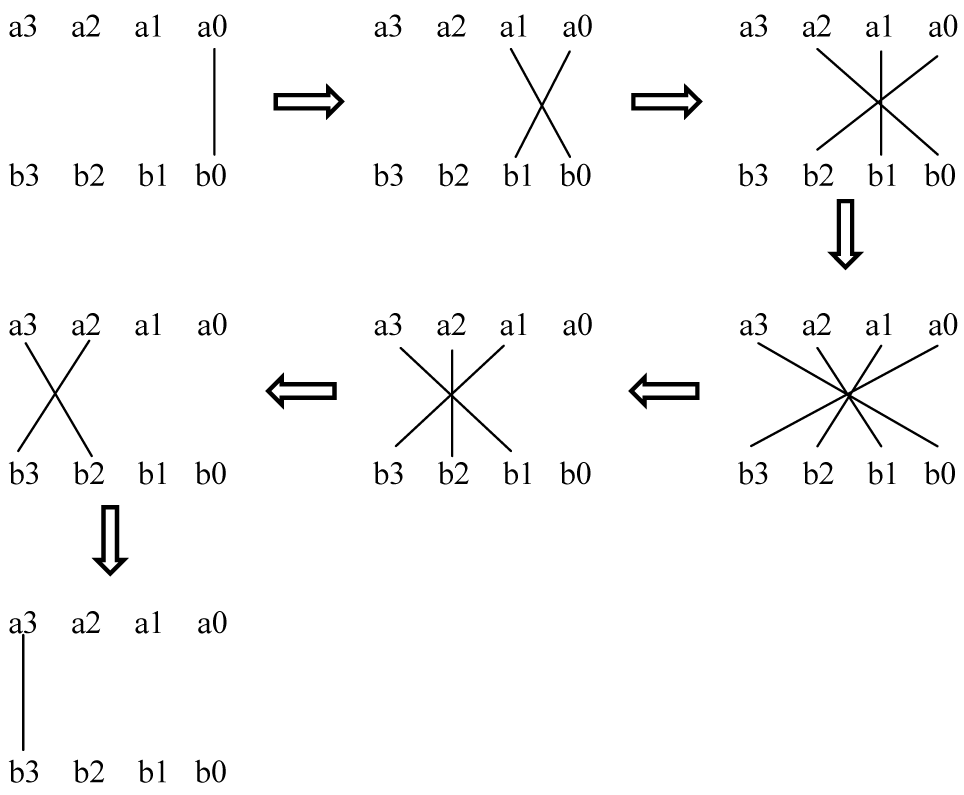##### Fig. 2. Hardware architecture of the proposed Vedic multiplier (2 $\textit{${\times}$}$ 2) using AND and $\textit{OR.}$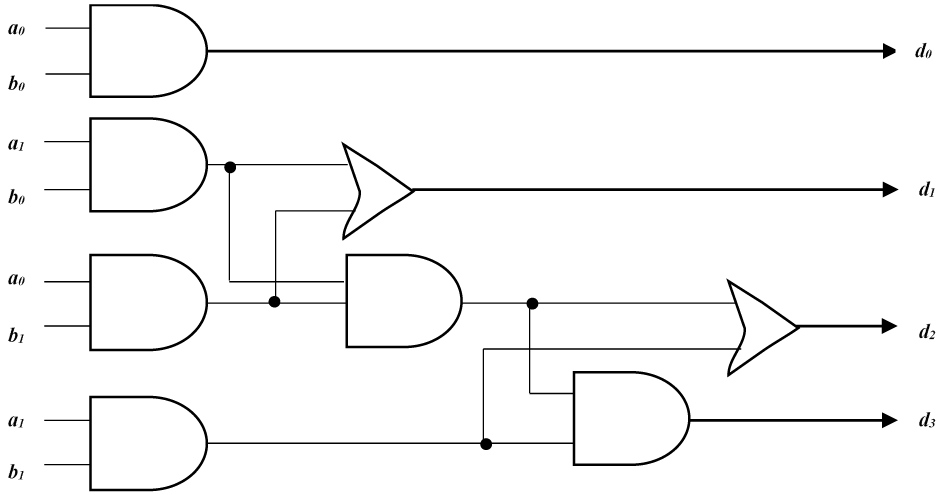$\textbf{Algorithm 2}$ Vedic Multiplier

$\textbf{Inputs:}$ A = a$_{3}$a$_{2}$a$_{1}$a$_{0}$

B = b$_{3}$b$_{2}$b$_{1}$b$_{0}$

$\textbf{Output:}$ P

 $\textbf{begin}$

 d$_{0}$$\textit{= a}$$_{0}$$\textit{{\times}b}$$_{0}$

 c$_{1}$d$_{1}$$\textit{= a}$$_{0}$$\textit{{\times}b}$$_{1}$$\textit{+a}$$_{1}$$\textit{{\times}b}$$_{0}$

 c$_{2}$d$_{2}$$\textit{= a}$$_{0}$$\textit{{\times}b}$$_{2}$$\textit{+a}$$_{1}$$\textit{{\times}b}$$_{1}$$\textit{+a}$$_{2}$$\textit{{\times}b}$$_{0}$\textit{+c}$_{1}$

 c$_{3}$d$_{3}$$\textit{= a}$$_{0}$$\textit{{\times}b}$$_{3}$$\textit{+a}$$_{1}$$\textit{{\times}b}$$_{2}$$\textit{+a}$$_{2}$$\textit{{\times}b}$$_{1}$\textit{+ a}$_{3}$$\textit{{\times}b}$$_{0}$\textit{+c}$_{2}$

 c$_{4}$d$_{4}$$\textit{= a}$$_{1}$$\textit{{\times}b}$$_{3}$$\textit{+a}$$_{2}$$\textit{{\times}b}$$_{2}$$\textit{+a}$$_{3}$$\textit{{\times}b}$$_{1}$\textit{+c}$_{3}$

 c$_{5}$d$_{5}$$\textit{= a}$$_{2}$$\textit{{\times}b}$$_{3}$$\textit{+a}$$_{3}$$\textit{{\times}b}$$_{2}$\textit{+c}$_{4}$

 c$_{6}$d$_{6}$$\textit{= a}$$_{3}$$\textit{{\times}b}$$_{3}$ \textit{+ c}$_{5}$

 $\textbf{end}$

 $\textbf{return}$ P = c$_{6}$d$_{6}$d$_{5}$d$_{4}$d$_{3}$d$_{2}$d$_{1}$d$_{0}$

$\textbf{end}$

Here, the Least Significant Bit (LSB), d$_{0}$ is obtained by multiplying the LSB of the multiplicand and the multiplier. In the following step, the bits on both sides of the line (refer to Fig. 1) are multiplied. By this mechanism, we get one of the bits of the result (d$_{n}$) and a carry (c$_{n}$). This carry is added in the next step and hence the process is perpetuated. If more than one line is present in one step, all the results are added to the previous carry. In each step, the least significant bit acts as the result bit and all the other bits act as carry. This process continues until every individual bit is multiplied according to Fig. 1. Finally, all the obtained bit values produce the required product P.

The order to be followed in a Vedic multiplication method is random. Using this 4 $\textit{${\times}$}$ 4 multiplier mechanism recursively, 8 $\textit{${\times}$}$ 8, 16 $\textit{${\times}$}$ 16, and 32 $\textit{${\times}$}$ 32, and other higher bit multipliers can be designed. Fig. 2 shows the proposed hardware architecture of a 2 $\textit{${\times}$}$ 2 Vedic multiplier.

The proposed multiplier requires 14 ${\times}$ 14 and 15 ${\times}$ 15 Vedic multipliers. The hardware architecture consists of AND gates and OR gates for efficient computation and makes the multiplication mechanisms easier for lower-order bits. In our proposed method we have used only OR gates and AND gates instead of XOR gates. This is because OR gates require lesser slices than XOR gates. This can be proven using their corresponding NAND equivalent. OR gate equals 3 NAND gates and XOR takes 4 NAND gates thus making the usage of lesser resources for our processor. Hence, we say that our method uses lesser gates thus making our processor occupy a lesser area.

For a 233-bit scalar multiplication, the bits are divided into two halves and given to the Karatsuba algorithm. This in turn is divided into two halves and given to simple Karatsuba multiplication. This is followed until the bits are reduced to lower degrees. This method is used for the higher-order bits as it uses a lesser number of AND and XOR gates. The initial multiplication for higher-order is done using the Karatsuba multiplier and the final multiplication for 14 ${\times}$14 and 15 ${\times}$ 15 bits are done using the designed Vedic multiplier.

##### Table 1 Slices occupied by the proposed ECC processor and comparison of multipliers
 ECC PROCESSOR PLATFORM SLICES Slices occupied by our ECC Processor KS_V Virtex-4 17505 Virtex-7 19489 Zynq 7000 19489 Slices occupied by Hybrid KS  and KS_V multiplier Hybrid KS Virtex-4 10434 Virtex-7 15530 Zynq 7000 15054 KS_V Virtex-4 9317 Virtex-7 10760 Zynq 7000 10760
##### Fig. 3. KS_V Multiplier Design Flow.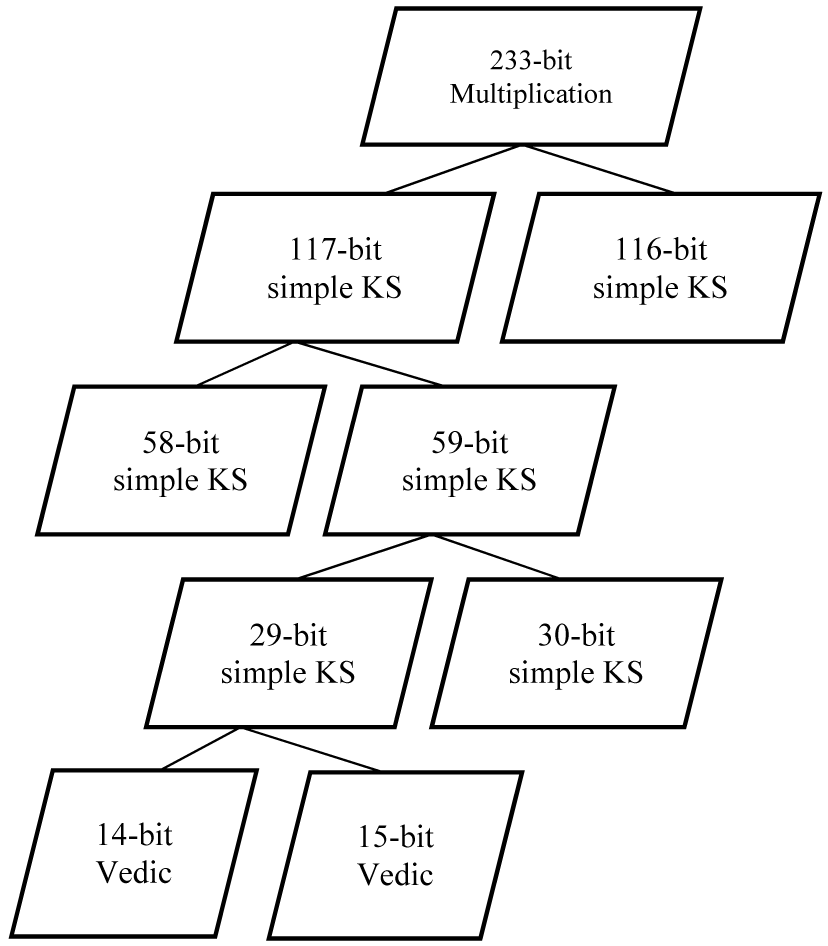Fig. 3 shows the design flow of our KS_V multiplier consisting of the Karatsuba multiplier and our Vedic multiplier design. The higher-order multiplication method follows the simple Karatsuba algorithm and the lowest order multiplication is done using the Vedic multiplier. As the concept of the Karatsuba algorithm is to use the divide and conquer approach, it initially breaks the inputs into the most significant half and least significant half. This algorithm is best suited for operands of higher bit length and loses its efficacy at lower bit lengths. Hence, the Vedic Algorithm is followed at lower bit multiplications since the binary multiplication is best suited in terms of area and delay for lower-order bits when compared to higher-order bits. When the order of bits is increased in Vedic multiplication it results in delay. These constraints of Karatsuba and Vedic Algorithms are taken as an advantage in the KS_V multiplier design and hence the multiplier becomes more efficient.

## V. IMPLEMENTATION RESULTS AND ANALYSIS

The verilog hardware description language model of the proposed architecture is created to verify and analyze the performance of the proposed design. The proposed architecture is synthesized, placed, and routed on three Xilinx platforms such as Xilinx Virtex-4 (xc4vfx 12-12sf363) device using Xilinx ISE 14.7, Xilinx Virtex 7 (xc7vx485tffg 1761-2) device, and ZedBoard (xc7z020clg484-1) using Vivado 2018.2.

The proposed Vedic multiplier was synthesized in Xilinx ISE and the RTL schematic was obtained. Our Vedic design occupied 82 slices for 14 $\textit{${\times}$}$ 14 bits and 94 slices for 15 $\textit{${\times}$}$ 15 bits which are lesser compared to other designs [1,14]. Only 16 AND gates and 9 OR gates were used for a basic 4 $\textit{${\times}$}$ 4 Vedic multiplication proving that our Vedic Multiplication design is area efficient. The implementation results of the proposed ECC processor using the KS_V multiplier and the comparison between the slices occupied by the proposed multiplier and hybrid KS multiplier are shown in Table 1.

The ECC processor using the hybrid Karatsuba multiplier  is compared with our proposed ECC architecture. The obtained results are shown in Table 2. Moreover, our proposed ECC architecture occupied 37 % LUT, 8 % LUTRAM, 1 % FF, 5 % IO and 3% BUF. Different Karatsuba multipliers are taken as a reference to check the number of slices occupied. Fig. 5 shows the area occupied by the existing Karatsuba multipliers  and our multiplier in Virtex 4 platform. From the above results, it is obvious that our proposed multiplier (KS_V) occupies a lesser area when compared to the previously existing work . This is because our Vedic multiplier design has lesser computational overhead and when combined with a simple Karatsuba multiplier gives lesser slices.

Since ECC is a lightweight algorithm, the area efficiency is an important parameter to be noted while implementing in a hardware device (OBU of a vehicle). To achieve this, our KS_V multiplier is implemented for scalar multiplication which is found to be occupying a comparatively lesser number of slices. This in turn proves that designing a lighter multiplier to occupy lesser slices is always cost-efficient and area efficient.

##### Table 2 Comparison of slices occupied byand our processor
 ECC PROCESSOR PLATFORM SLICES HKS  Virtex-4 19304 Virtex-7 22832 Zynq 7000 22536 KS_V Virtex-4 17505 Virtex-7 19489 Zynq 7000 19489
##### Fig. 5. Slices occupied by the multipliers in Xilinx Virtex 4.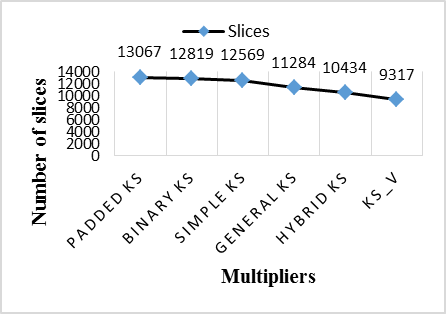##### Table 3 Slices Comparison
 REF FIELD MULTIPLIER SLICES  GF (2233) GNB Mul 16782  GF (2233) Digit-Serial GF Mul 16766  GF (2233) Hybrid Bit parallel KS Field Mul 13620  GF (2233) Bit-Serial Mul 15209  GF (2233) Digit-Serial Systolic KS Mul 22716 proposed GF (2233) KS_V Mul 10760

Table 3 provides a comparison between the different multipliers used and the number of slices occupied by each multiplier. The GNB multiplier used in  is based on exponentiation by powers of 2 and multiplication by the normal element of GF(2$^{m}$) and is said to have low hardware complexity and low critical path delay. The design is based upon the usage of some regular modules for computation of exponentiation by powers of 2 and low-cost blocks for multiplication by normal elements of the binary field. For the powers of 2 exponents, the modules are implemented by some simple cyclic shifts in the normal basis representation.

The Digit-Serial GF Multiplier  uses different levels of digit-serial computation were applied to the data path of Galois field multiplication and division to explore the resulting performances and find out an optimal digit size. More resources were used to achieve faster circuits. The multiplier was designed using three multipliers and one divider. The Hybrid bit-parallel Karatsuba field multiplier (HBKM)  has the sub-quadratic complexity of the Karatsuba algorithm coupled with efficient utilization of the FPGA’s LUT resources. Moreover, this scheme requires lesser clock cycles. The HBKM operates by splitting the input operands up to a threshold and then applying threshold multipliers. The outputs of the threshold multipliers are combined and then reduced.

In the Bit serial Multiplier  each point operation is decomposed to its basic GF(2$^{k}$) operations. The GF(2$^{k}$) operations that can be performed in parallel are identified and are multiplexed with similar operations in the consecutive point operation in the PM algorithm so as to maximize parallelism. Using this approach, two different PM accelerator architectures using bit-serial or bit-parallel GF(2$^{k}$) multipliers were developed. In the Digit-Serial Systolic KS multiplier  a digit-serial structure has been derived, where three different approaches have been introduced to reduce the register-complexity. These digit-parallel structures were presented for FPGA platforms but can also be used in application-specific integrated circuit (ASIC) platforms for low power and high-performance implementation.

From this comparison, it is evident that our proposed multiplier shows better area efficiency. Our ECC processor is found to function at the time period of 0.060~ms, which consumes 1.545 W power and 92.7 $\textit{${\mathrm{\mu}}$J}$ energy. Since we have focused only on the area occupied, we have not included any comparison for the parameters mentioned above.

## VI. CONCLUSION

In this work, a novel multiplication method is proposed to reduce the area occupied by the multiplication algorithms during scalar multiplication. The proposed architecture is implemented in ZedBoard, Xilinx Virtex 7, and Virtex 4. ZedBoard is chosen as the prime target of our implementation as it supports certain distinguished functions and can be used in a wide range of applications. Since it has a tightly coupled ARM processing system and 7-series programmable logic, it can be used to create unique and powerful designs with the ZedBoard. Because of this property, the above implementation can be targeted in On-Board Units (OBU) in vehicles. This is mainly due to the lesser area occupied by the ECC processor.

## References

1
Chester Rebeiro , Debdeep Mukhopadhyay , 2007, Hybrid Masked Karatsuba Multiplier For Gf(2$^{233}$), Proceedings of the 11th IEEE VLSI Design and Test Symposium, Kolkata, VLSI Society of India., No. 1, pp. 379-3872
Ravi Kishore Kodali , Siva Kumar , Vishal Jain , Boppana Lakshmi , December 2015, Hybrid Multiplier for ECC, IEEE Recent Advances in Intelligent Computational Systems (RAICS), pp. 65-703
Kadu Rakesh K., Adane Dattatraya S., August 2019, Hardware Implementation of Efficient Elliptic Curve Scalar Multiplication using Vedic Multiplier, International Journal of Communication Networks and Information Security (IJCNIS), Vol. 11, No. 2, pp. 270-277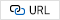4
Steffen Peter , Peter LangendOrfer , 2007, An Efficient Polynomial Multiplier in GF(2m) and its Application to ECC Designs, Design, Automation & Test in Europe Conference & Exhibition, Nice, pp. 1-65
Jiafeng Xie , Pramod Kumar Meher , Mingui Sun , Yuecheng Li , Bo Zeng , Zhi-Hong Mao , July 2017, Efficient FPGA Implementation of Low-Complexity Systolic Karatsuba Multiplier Over GF(2m) Based on NIST Polynomials, IEEE Transactions On Circuits And Systems-I: Regular Papers, Vol. 64, No. 7, pp. 1815-18256
Chiou-Yng Lee , Chia-Chen Fan , Jiafeng Xie , Shyan-Ming Yuan , 2018, Efficient Implementation of Karatsuba Algorithm Based Three-Operand Multiplication Over Binary Extension Field, IEEE Access, Vol. 6, pp. 38234-382427
Bonifus , Dani George , 2013, Ecc Encryption System Using Encoded Multiplier And Vedic Mathematics., International Journal of Advanced Research in Electrical, Electronics and Instrumen-tation Engineering8
Manoj Kumar , Ankur Kumar , August 2019, Some Algorithms of Various Projective Coordinate Systems for ECC Using Ancient Indian Vedic Mathematics Sutras, International Journal Of Scientific & Technology Research, Vol. 8, No. 089
Susantio, D , Muchtadi-Alamsyah, Intan , 2016, Implementation of Elliptic Curve Cryptography in Binary Field, Journal of Physics: Conference Series. 710. 012022.10
Nguyen N., Gaj K., Caliga D., El-Ghazawi T., Tokyo December 2003, Implementation of Elliptic Curve Cryptosystems on a Reconfigurable Computer, George Mason University, SRC Computers, The George Washington University. Second International Conference on Field-Programmable Technology, FPT 200311
Hankerson, Darrel , Vanstone, Scott , Menezes, Alfred , 2004, Guide to Elliptic Curve Cryptography, Springer Professional Computing. New York: Springer-Verlag, ISBN 0-387-95273-X.12
https://en.wikipedia.org/wiki/Elliptic_curve_point_multiplication#Point_addition13
Karatsuba Anatoly A., Ofman Y., 1963, Multiplication of Multidigit Numbers on Automata, Soviet Physics Doklady, Vol. 7, pp. 595-59614
Arish S., Sharma R. K., 2015, An efficient binary multiplier design for high speed applications using Karatsuba algorithm and Urdhva-Tiryagbhyam algorithm, 2015 Global Conference on Communication Technologies (GCCT), Thuckalay, pp. 192-19615
Rashidi B., Sayedi S. M., Rezaeian Farashahi R., 3 2017, Efficient and low-complexity hardware architecture of Gaussian normal basis multiplication over GF(2m) for elliptic curve cryptosystems, in IET Circuits, Devices & Systems, Vol. 11, No. 2, pp. 103-11216
Sutter G. D., Deschamps J., Imana J. L., Jan. 2013, Efficient Elliptic Curve Point Multiplication Using Digit-Serial Binary Field Operations, in IEEE Transactions on Industrial Electronics, Vol. 60, No. 1, pp. 217-22517
Rebeiro C., Roy S.S., Mukhopadhyay D., 2012, Pushing the Limits of High-Speed GF(2m) Elliptic Curve Scalar Multiplication on FPGAs., In: Prouff E., Schaumont P. (eds) Cryptographic Hardware and Embedded Systems-CHES 2012. CHES 2012. Lecture Notes in Computer Science, Springer, Berlin, Heidelberg, Vol. 742818
Fournaris A. P., Zafeirakis J., Koufopavlou O., 2014, Designing and Evaluating High Speed Elliptic Curve Point Multipliers, 2014 17th Euromicro Conference on Digital System Design, Verona, Vol. verona, No. 2014, pp. 169-17419
Chiou C.W., Lee Chiou-Yng, Lin J.-M., Yeh Y.-C., Chang H.W., Lin L.-C., 2015, Digit-serial systolic Karatsuba multiplier for special classes over GF(2m)., Journal of Computers (Taiwan), Vol. 26, pp. 40-57Renita J, has completed her Bachelor’s degree from St. Xaviers Catholic College of Engineering, Anna University in Electronics and Communication engineering in the year 2015. She obtained her Master’s degree from Sri Sivasubramaniya Nadar college of engineering, Anna University in Communication Systems in the year 2017. She is a part-time research scholar doing PhD in the area of Elliptic Curve Cryptogaphy at Anna University. She is currently working as Project Associate in Hardware Security Research Group at Society for Electronic transaction and security (SETS), Chennai.

Edna Elizabeth N, has graduated her Bachelor’s degree from Government college of Technology –Coimbatore in Electronics and communication in the year 1990. She obtained her Master’s degree from MIT-Anna University in the year 2001. She completed her Ph.D in the area of Mobile Ad hoc networking at Anna University. She has 25 years of teaching experience and has been working for a reputed company for 5 years. She is currently working as Professor in Electronics and communication department in Sri Sivasubramaniya Nadar college of engineering, SSN Nagar, kalavakkam, Chennai 603 110, India. She has 55 publications in International and National Journals and conferences in the area of Mobile Ad hoc Networks. Her current area of research is security issues in Mobile Ad hoc Networks and Vehicular Ad hoc Networks. She received Best Faculty award for the outstanding performance for the academic year 2006-2007 & 2009-2010 at SSN.

Nandhini Asokan, has completed her Bachelor’s degree from Sri Krishna College of Engineering and Technology, Coimbatore. She obtained her Master’s degree from Sri Sivasubramaniya Nadar college of engineering, Anna University in VLSI in the year 2020. She is currently working as Associate System Engineer at Tata Consultancy Services (TCS), Chennai.